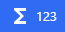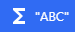# Expressions

When adding a condition or an action to an event, some fields have the following icons alongside them:These icons indicate that the field accepts an expression. An expression is a formula, like in mathematics, that can be composed of:

Tip

For a complete list of the function expressions that GDevelop provides out of the box, refer to expressions reference.

## Numbers

When the following icon appears alongside a field, the field accepts a numeric expression:The following values are examples of numeric expressions, composed of a single number:

• `0`
• `-10`
• `25.5`

You can also use mathematical operators to add, subtract, multiply, and divide numbers:

• `2+2`
• `3-1`
• `10*10`
• `99/3`

This is the complete list of operators:

• `+` (add)
• `-` (subtract)
• `*` (multiply)
• `/` (divide)

Finally, functions can be used - see the next sections.

## Text

When the following icon appears alongside a field, the field accepts a text expression:The following values are examples of text expressions, composed of a single text:

• `"Hello world"`
• `"This is a text expression"`
• `"GDevelop is cool!"`

All texts must be wrapped in double quotes. Without the double quotes, GDevelop assumes the value is a function or a variable.

You can use the `+` operator to concatenate two or more strings of text. For example, `"Hello" + "World"` becomes `"HelloWorld"`. The other operators are not valid when working with text (it does not make sense to subtract, multiply or divide a text by another).

## Variables (or properties)

In an expression, you can write the name of a variable (a scene variable, a global variable). For example, if a variable called Score was added to the scene, you can simply write: `2 * Score` to compute the score value multiplied by two.

You can also access to the value of a variable of an object by writing the object name followed by a dot and the name of the variable. For example: `Player.Ammo` or `Player.CoinEarned`.

Finally, when you're editing a behavior or a custom object inside an extension, you can also access the properties of this behavior or object by writing their name, like variables.

Note

You can also use the functions `Variable(<name of the variable>)` (for variables containing a number) and `VariableString(<name of the variable>)` (for variables containing a string) to access the value stored inside a scene variable. There are also functions to access global variables (`GlobalVariable(<name of the variable>)`) and object variables (`Object.Variable(<name of the variable>)`).

These functions are more verbose and more cumbersome to use. Most of the time, you can just write the name of the variable you want to use.

## Functions

Often, it's necessary to calculate complex values using data coming from the game. For example, you might want to compute something like a score according to the X and Y coordinates of the player.

This is where functions are useful.

You can use functions to dynamically calculate values while the game is running. For example, the `X` and `Y` functions calculate the coordinates of an object:

``````ObjectName.X()
ObjectName.Y()
``````

Using a function is often referred to as calling a function.

Tip

If you're familiar with formulas in spreadsheets, functions in programming languages, or functions in mathematics, functions in GDevelop are the same thing.

### Syntax

There are three types of functions:

• Functions without objects. For example: `cos(3.14)`.
• Functions with objects. For example: `Player.X()`.
• Functions with objects and behaviors. For example: `Player.Animation::Index()`.

#### Functions without objects

Some functions exist independently of objects and behaviors. You don't need any object or behavior to call these functions.

These are some examples of functions without objects:

• `CurrentSceneName()` - Get the name of the current scene.
• `FileSystem::DesktopPath()` - Get the path of the "Desktop" directory.
• `ToNumber(<number>)` - Convert a string into a number.

Note

The `::` syntax is used to group functions related to a same feature or extension. For example, all of the file system functions are prefixed with `FileSystem::`. This helps keep related functions organized. The `::` syntax does not change the behavior of the function.

#### Functions with objects

Some functions are called on objects. This means the function affects or retrieves data about a specific object.

These are some examples of functions that are used with objects. The placeholder `<object>` must be replaced with the name of an object in your game:

• `<object>.Angle()` - Get the angle of the object (in degrees).
• `<object>.Layer()` - Get the name of the layer that the object is on.
• `<object>.ObjectName()` - Get the name of the object.

#### Functions with objects and behaviors

Some functions are functions provided by a behavior attached to an object. This means the function affects or retrieves data about a specific behavior that is attached to an object. This is often useful to manipulate or read data from the behavior.

These are some examples of functions with objects and behaviors:

• `<object>.Pathfinding::Speed()`
• `<object>.Physics2::Friction()`
• `<object>.PlatformerObject::Gravity()`

Note

You can see again the `::` syntax here. This separate the behavior name and the name of the function. This is useful in case you have multiple behaviors on a single object.

### Arguments

Some functions accept arguments. An argument is a value that:

• can be passed into a function
• affects the output of the function

For example, the `ToString` function converts a number into a string, but it can't do anything unless you provide it with a number. That number is the argument. In the following example, the number `42` is the argument:

``````ToNumber(42)
``````

Some functions, such as the `RandomInRange` function, accept multiple arguments:

``````RandomInRange(3, 10)
``````

When a function accepts multiple arguments, each argument must be separated by a comma.

### Return values

When a function calculates a value, it's said to return that value. This value is the output of the function. For example, if the X coordinate of an object is `42`, the `ObjectName.X()` function returns `42`. The value returned by a function depends on the object, the arguments, and the state of the game.

Functions can return numbers or strings. The type of value a function returns determines whether or not a function can be used in a field. For example, the `ToString` function returns a string, so it can't be used in a field that expects a number, while the `ToNumber` function returns a number, so it can't be used in a field that expects a string.